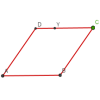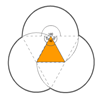#### You may also like### Just Rolling Round

P is a point on the circumference of a circle radius r which rolls, without slipping, inside a circle of radius 2r. What is the locus of P?### Roaming Rhombus

We have four rods of equal lengths hinged at their endpoints to form a rhombus ABCD. Keeping AB fixed we allow CD to take all possible positions in the plane. What is the locus (or path) of the point D?### Triangles and Petals

An equilateral triangle rotates around regular polygons and produces an outline like a flower. What are the perimeters of the different flowers?

# Rolling Coins

##### Age 14 to 16 Challenge Level:

When coin A rolls once all the way around coin B (which is stationary) then coin A makes two revolutions. No-one came up with a satisfactory reason why this should be. Is it really so counter intuitive? Perhaps you may like to consider points at the centre and circumference of coin A as it rolls through a specific arc around coin B.

However, Thomas from Madras College, St Andrews had convinced himself that 2 is the correct solution because "as it travels around the stationary coin the distance it goes accounts for 1 revolution and as it moves around it is rotating so it ends up putting in an extra revolution".

He built upon this insight, as well as that concerning the centres of the coins involved, to generalise what happens when a coin rolls around a line of 'n' similar coins.

When the rolling coin rolls around two stationary coins it revolves through an angle of 16($\pi$/3) or 8/3 revolutions.

The number of revolutions R, when n is the number of stationary identical coins, is given by the formula:

R = 2(n+2)/3.